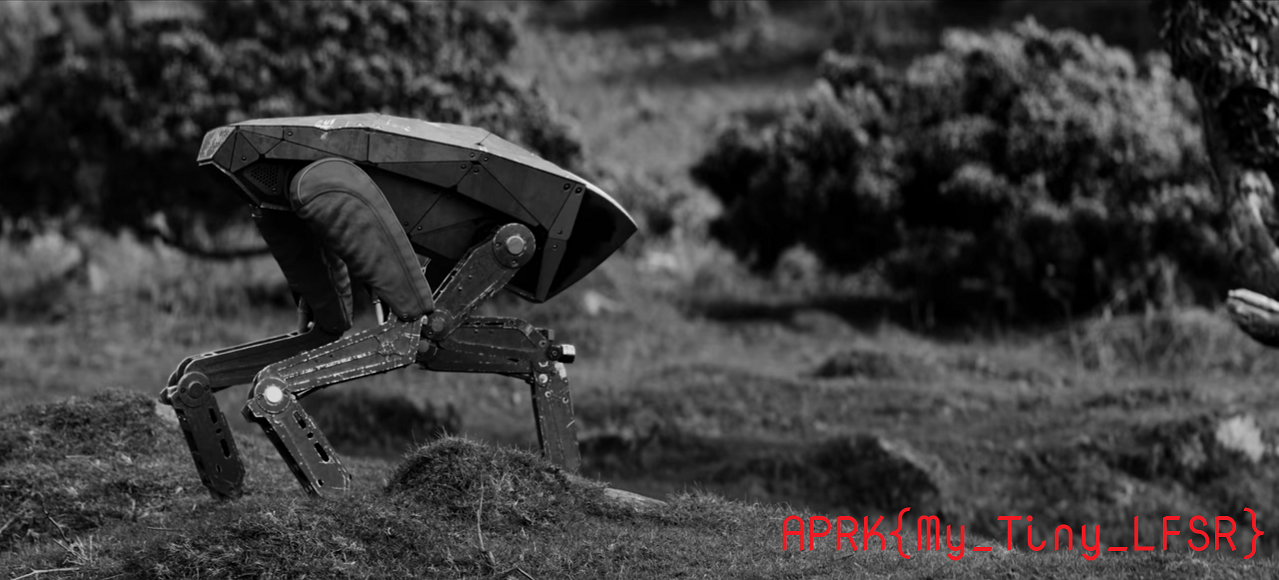# Robot Cipher

Aperi'CTF 2019 - Hardware (175 pts).

# Aperi’CTF 2019 - Robot Cipher

### Challenge details

Event Challenge Category Points Solves
Aperi’CTF 2019 Robot Cipher Hardware 175 2

L’entreprise “MetalHead” utilise une solution de chiffrement hardware “Next Gen”. Vous avez été mandaté pour vérifier la robustesse de cette solution.

Challenge:

• Robot.png - md5sum: 9be15749b7c8276113452dbefd4a154a
• Cipher - md5sum: e44b002660a92efd5fe555b0dc7999ce

### TL;DR

Robot.png was an image of a LFSR (linear feedback shift register) and Cipher was a xored file with the LFSR stream.

### Methodology

#### Understand the image

First, let’s have a look to the Robot.png file:We can see a “loop” with 16 registers on the bottom, and gates at the top. This image looks like a Linear Feedback Shift Register (LFSR) which is a Pseudo-Random Number Generator (PRNG). By looking closer, we can get the initial state for each register: 0,0,0,0,0,1,0,0,0,0,1,0,0,1,0,0.

#### Code the LFSR

To get the LFSR stream, I decided to re-code it in Python:

#!/usr/bin/env python3

import sys
assert sys.version_info == 3  # ==> Run in Python3 only ;)

outbin = ''
for i in range(100):  # get 100 bits
new = r ^ int(not (int(not r) ^ ( r ^ r )))  # Next step
outbin += str(r[-1])  # Get output
r = [new]+r[:-1] # Update registers

print(outbin)  # print 100 bits from LFSR stream


#### XOR with file

Now we need to decipher the file. We’ll use the most common “simple” way to cipher with a stream: the xor operation. Here is the final Python script:

#!/usr/bin/env python3

import sys
assert sys.version_info == 3  # ==> Run in Python3 only ;)

input = r"Cipher"
output = r"Cipher.png"

r = [0,0,0,0,0,1,0,0,0,0,1,0,0,1,0,0] # registres

with open(input,"rb") as f:
binary = ''.join([bin(l)[2:].zfill(8) for l in f.read()])  # File in "binary"

outbin = ''
for b in binary:
new = r ^ int(not (int(not r) ^ ( r ^ r )))  # Next step
outbin += str(int(b) ^ r[-1])  # Xor with output bit
r = [new]+r[:-1] # Update registers

out = bytes([int(outbin[z:z+8],2) for z in range(0,len(outbin),8)])  # "binary" to bytes

with open(output,"wb") as f:
f.write(out)


And here is the output file (a PNG file):#### Flag

APRK{My_Tiny_LFSR}

Zeecka